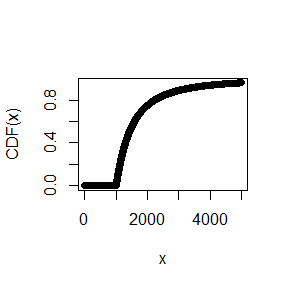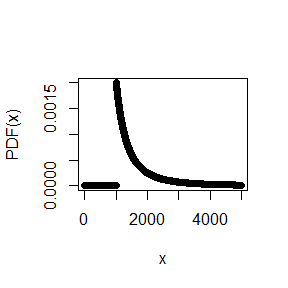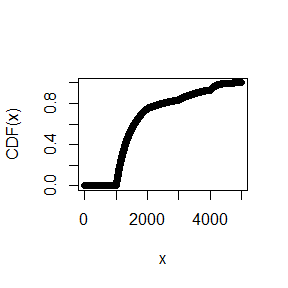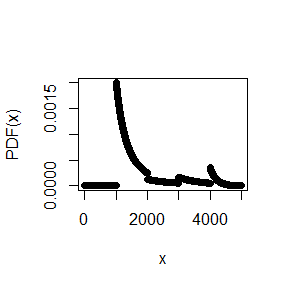# Pareto Package Vignette

## Introduction

The (European) Pareto distribution is probably the most popular distribution for modeling large losses in reinsurance pricing. There are good reasons for this popularity, which are discussed in detail in Fackler (2013). We recommend Philbrick (1985) and Schmutz et.al. (1998) for an impression of how the (European) Pareto distribution is applied in practice.

In cases where the Pareto distribution is not flexible enough, pricing actuaries sometimes use piecewise Pareto distributions. For instance, a Pareto alpha of 1.5 is used to model claim sizes between USD 1M and USD 5M and an alpha of 2.5 is used above USD 5M. A particularly useful and non-trivial application of the piecewise Pareto distribution is that it can be used to match a tower of expected layer losses with a layer independent collective loss model. Details are described in Riegel (2018), who also provides a matching algorithm that works for an arbitrary number of reinsurance layers.

The package provides a tool kit for the Pareto and the Piecewise Pareto distribution, which is useful for pricing of reinsurance treaties. In particular, the package provides the matching algorithm for layer losses.

## Pareto distribution

Definition: Let $$t>0$$ and $$\alpha>0$$. The Pareto distribution $$\text{Pareto}(t,\alpha)$$ is defined by the distribution function $F_{t,\alpha}(x):=\begin{cases} 0 & \text{ for x\le t} \\ \displaystyle 1-\left(\frac{t}{x}\right)^{\alpha} & \text{ for x>t.} \end{cases}$ This version of the Pareto distribution is also known as Pareto type I, European Pareto or single-parameter Pareto.

### Distribution function and density

The functions pPareto and dPareto provide the distribution function and the density function of the Pareto distribution:

library(Pareto)
x <- c(1:10) * 1000
pPareto(x, 1000, 2)
##   0.0000000 0.7500000 0.8888889 0.9375000 0.9600000 0.9722222 0.9795918
##   0.9843750 0.9876543 0.9900000
plot(pPareto(1:5000, 1000, 2), xlab = "x", ylab = "CDF(x)")dPareto(x, 1000, 2)
##   0.000000e+00 2.500000e-04 7.407407e-05 3.125000e-05 1.600000e-05
##   9.259259e-06 5.830904e-06 3.906250e-06 2.743484e-06 2.000000e-06
plot(dPareto(1:5000, 1000, 2), xlab = "x", ylab = "PDF(x)")The package also provides the quantile function:

qPareto(0:10 / 10, 1000, 2)
##   1000.000 1054.093 1118.034 1195.229 1290.994 1414.214 1581.139
##   1825.742 2236.068 3162.278      Inf

### Simulation:

rPareto(20, 1000, 2)
##   1069.883 1226.808 1070.066 2441.235 1727.387 1197.475 1410.243
##   1426.691 1493.052 1264.147 1156.218 1061.947 1733.017 1283.406
##  1433.116 1351.829 1054.235 1003.015 1189.447 1208.854

### Layer mean:

Let $$X\sim \text{Pareto}(t,\alpha)$$ and $$a, c\ge 0$$. Then $E(\min[c,\max(X-a,0)]) = \int_a^{c+a}(1-F_{t,\alpha}(x))\, dx =: I_{t,\alpha}^{\text{c xs a}}$ is the layer mean of $$c$$ xs $$a$$, i.e. the expected loss to the layer given a single loss $$X$$.

Example: $$t=500$$, $$\alpha = 2$$, Layer 4000 xs 1000

Pareto_Layer_Mean(4000, 1000, 2, t = 500)
##  200

### Layer variance:

Let $$X\sim \text{Pareto}(t,\alpha)$$ and $$a, c\ge 0$$. Then the variance of the layer loss $$\min[c,\max(X-a,0)]$$ can be calculated with the function Pareto_Layer_Var.

Example: $$t=500$$, $$\alpha = 2$$, Layer 4000 xs 1000

library(Pareto)
Pareto_Layer_Var(4000, 1000, 2, t = 500)
##  364719

### Pareto extrapolation

Consider two layers $$c_i$$ xs $$a_i$$ and a $$\text{Pareto}(t,\alpha)$$ distributed severity with sufficiently small $$t$$. What is the expected loss of $$c_2$$ xs $$a_2$$ given the expected loss of $$c_1$$ xs $$a_1$$?

Example: Assume $$\alpha = 2$$ and the expected loss of 4000 xs 1000 is 500. Calculate the expected loss of the layer 5000 xs 5000.

Pareto_Extrapolation(4000, 1000, 5000, 5000, 2) * 500
##  62.5
Pareto_Extrapolation(4000, 1000, 5000, 5000, 2, ExpLoss_1 = 500)
##  62.5

### Pareto alpha between two layers:

Given the expected losses of two layers, there is typically a unique Pareto alpha $$\alpha$$ which is consistent with the ratio of the expected layer losses.

Example: Expected loss of 4000 xs 1000 is 500. Expected loss of 5000 xs 5000 is 62.5. Alpha between the two layers:

Pareto_Find_Alpha_btw_Layers(4000, 1000, 500, 5000, 5000, 62.5)
##  2

Check: see previous example

### Pareto alpha between a frequency and layer:

Given the expected excess frequency at a threshold and the expected loss of a layer, then there is typically a unique Pareto alpha $$\alpha$$ which is consistent with this data.

Example: Expected frequency in excess of 500 is 2.5. Expected loss of 4000 xs 1000 is 500. Alpha between the frequency and the layer:

Pareto_Find_Alpha_btw_FQ_Layer(500, 2.5, 4000, 1000, 500)
##  2

Check:

Pareto_Layer_Mean(4000, 1000, 2, t = 500) * 2.5
##  500

### Matching the expected losses of two layers:

Given the expected losses of two layers, we can use these techniques to obtain a Poisson-Pareto model which matches the expected loss of both layers.

Example: Expected loss of 30 xs 10 is 26.66 (Burning Cost). Expected loss of 60 xs 40 is 15.95 (Exposure model).

Pareto_Find_Alpha_btw_Layers(30, 10, 26.66, 60, 40, 15.95)
##  1.086263

Frequency @ 10:

26.66 / Pareto_Layer_Mean(30, 10, 1.086263)
##  2.040392

A collective model $$\sum_{n=1}^NX_n$$ with $$X_N\sim \text{Pareto}(10, 1.09)$$ and $$N\sim \text{Poisson}(2.04)$$ matches both expected layer losses.

### Frequency extrapolation and alpha between frequencies:

Given the frequency $$f_1$$ in excess of $$t_1$$ the frequency $$f_2$$ in excess of $$t_2$$ can directly be calculated as follows: $f_2 = f_1 \cdot \left(\frac{t_1}{t_2}\right)^\alpha$ Vice versa, we can calculate the Pareto alpha, if the two excess frequencies $$f_1$$ and $$f_2$$ are given: $\alpha = \frac{\log(f_2/f_1)}{\log(t_1/t_2)}.$

Example:

Expected frequency excess 1000 is 2. What is the expected frequency excess 4000 if we have a Pareto alpha of 2.5?

t_1 <- 1000
f_1 <- 2
t_2 <- 4000
(f_2 <- f_1 * (t_1 / t_2)^2.5)
##  0.0625

Vice versa:

Pareto_Find_Alpha_btw_FQs(t_1, f_1, t_2, f_2)
##  2.5

### Maximum likelihood estimation of the parameter alpha

For $$i=1,\dots,n$$ let $$X_i\sim \text{Pareto}(t_i,\alpha)$$ be Pareto distributed observations. Then we have the ML estimator $\hat{\alpha}^{ML}=\frac{n}{\sum_{i=1}^n\log(X_i/t_i)}.$ Example:

Pareto distributed losses with a reporting threshold of $$t=100$$ and $$\alpha = 2$$:

losses <- rPareto(1000, t = 100, alpha = 2)
Pareto_ML_Estimator_Alpha(losses, t = 100)
##  1.972281

### Truncation

Let $$X\sim \text{Pareto}(t,\alpha)$$ and $$T>t$$. Then $$X|(X>T)$$ has a truncated Pareto distribution. The Pareto functions mentioned above are also available for the truncated Pareto distribution.

## Piecewise Pareto distribution

Definition: Let $$\mathbf{t}:=(t_1,\dots,t_n)$$ be a vector of thresholds with $$0<t_1<\dots<t_n<t_{n+1}:=+\infty$$ and let $$\boldsymbol\alpha:=(\alpha_1,\dots,\alpha_n)$$ be a vector of Pareto alphas with $$\alpha_i\ge 0$$ and $$\alpha_n>0$$. The piecewise Pareto distribution} $$\text{PPareto}(\mathbf{t},\boldsymbol\alpha)$$ is defined by the distribution function $F_{\mathbf{t},\boldsymbol\alpha}(x):=\begin{cases} 0 & \text{ for x<t_1} \\ \displaystyle 1-\left(\frac{t_{k}}{x}\right)^{\alpha_k}\prod_{i=1}^{k-1}\left(\frac{t_i}{t_{i+1}}\right)^{\alpha_i} & \text{ for x\in [t_k,t_{k+1}).} \end{cases}$

The family of piecewise Pareto distributions is very flexible:

Proposition: The set of Piecewise Pareto distributions is dense in the space of all positive-valued distributions (with respect to the Lévy metric).

This means that we can approximate any positive valued distribution as good as we want with piecewise Pareto. A very good approximation typically comes at the cost of many Pareto pieces. Piecewise Pareto is often a good alternative to a discrete distribution, since it is much better to handle!

The Pareto package also provides functions for the piecewise Pareto distribution. For instance:

### Distribution function

x <- c(1:10) * 1000
t <- c(1000, 2000, 3000, 4000)
alpha <- c(2, 1, 3, 20)
pPiecewisePareto(x, t, alpha)
##   0.0000000 0.7500000 0.8333333 0.9296875 0.9991894 0.9999789 0.9999990
##   0.9999999 1.0000000 1.0000000
plot(pPiecewisePareto(1:5000, t, alpha), xlab = "x", ylab = "CDF(x)")### Density

dPiecewisePareto(x, t, alpha)
##   0.000000e+00 1.250000e-04 1.666667e-04 3.515625e-04 3.242592e-06
##   7.048328e-08 2.768239e-09 1.676381e-10 1.413089e-11 1.546188e-12
plot(dPiecewisePareto(1:5000, t, alpha), xlab = "x", ylab = "PDF(x)")### Simulation

rPiecewisePareto(20, t, alpha)
##   1154.620 1527.895 1478.414 1579.710 1327.515 1236.439 1075.098
##   1321.882 1738.836 4103.994 1850.759 1642.031 1774.668 1670.843
##  3852.271 1051.340 1845.775 3890.973 1378.889 1132.315

### Layer mean

PiecewisePareto_Layer_Mean(4000, 1000, t, alpha)
##  826.6969

### Layer variance

PiecewisePareto_Layer_Var(4000, 1000, t, alpha)
##  922221.2

### Maximum likelihood estimation of the alphas

Let $$\mathbf{t}:=(t_1,\dots,t_n)$$ be a vector of thresholds and let $$\boldsymbol\alpha:=(\alpha_1,\dots,\alpha_n)$$ be a vector of Pareto alphas. For $$i=1,\dots,n$$ let $$X_i\sim \text{PPareto}(\mathbf{t},\boldsymbol\alpha)$$. If the vector $$\mathbf{t}$$ is known, then the parameter vector $$\boldsymbol\alpha$$ can be estimated with maximum likelihood.

Example:

Piecewise Pareto distributed losses with $$\mathbf{t}:=(100,\,200,\, 300)$$ and $$\boldsymbol\alpha:=(1,\, 2,\, 3)$$:

losses <- rPiecewisePareto(10000, t = c(100,200,300), alpha = c(1,2,3))
PiecewisePareto_ML_Estimator_Alpha(losses, c(100,200,300))
##  1.017926 2.027558 2.902659

### Truncation

The package also provides truncated versions of the piecewise Pareto distribution. In most functions there are two options available:

• truncation_type = 'lp': Below the largest threshold $$t_n$$, the distribution function equals the distribution of the piecewise Pareto distribution without truncation. The last Pareto piece, however, is truncated at truncation
• truncation_type = 'wd': The whole piecewise Pareto distribution is truncated at truncation’

## Matching a tower of layer losses

The Pareto distribution can be used to build a collective model which matches the expected loss of two layers. We can use piecewise Pareto if we want to match the expected loss of more than two layers.

Consider a sequence of attachment points $$0 < a_1 <\dots < a_n<a_{n+1}:=+\infty$$. Let $$c_i:=a_{i+1}-a_i$$ and let $$e_i$$ be the expected loss of the layer $$c_i$$ xs $$a_i$$. Moreover, let $$f_1$$ be the expected frequency in excess of $$a_1$$.

The following matching algorithm uses one Pareto piece per layer and is straight forward:

• Calculate the Pareto alpha $$\alpha_1$$ between the excess frequency $$f_1$$ and the layer $$c_1$$ xs $$a_1$$
• Calculate the frequency $$f_2$$ in excess of $$a_2$$: $$f_2:=(a_1/a_2)^{\alpha_1}\cdot f_1$$
• Calculate the Pareto alpha $$\alpha_2$$ between the excess frequency $$f_2$$ and the layer $$c_2$$ xs $$a_2$$
• Calculate the frequency $$f_3$$ in excess of $$a_3$$: $$f_3:=(a_2/a_3)^{\alpha_2}\cdot f_3$$
• $$\dots$$
• Use a collective model $$\sum_{n=1}^NX_n$$ with $$E(N)=f_1$$ and $$X_n\sim\text{PPareto}(\mathbf{t},\boldsymbol\alpha)$$.

This approach always works for three layers, but it often does not work if we have three or more layers. For instance, Riegel (2018) shows that it does not work for the following example:

$$i$$ Cover $$c_i$$ Att. Pt. $$a_i$$ Exp. Loss $$e_i$$ Rate on Line $$e_i/c_i$$
1 500 1000 100 0.20
2 500 1500 90 0.18
3 500 2000 50 0.10
4 500 2500 40 0.08

The Pareto package provides a more complex matching approach that uses two Pareto pieces per layer. Riegel (2018) shows that this approach works for an arbitrary number of layers with consistent expected losses.

Example:

attachment_points <- c(1000, 1500, 2000, 2500, 3000)
exp_losses <- c(100, 90, 50, 40, 100)
fit <- PiecewisePareto_Match_Layer_Losses(attachment_points, exp_losses)
fit
## $t ##  1000.000 1500.000 1932.059 2000.000 2147.531 2500.000 2847.756 3000.000 ## ##$alpha
##  0.3091209 0.1753613 9.6851892 3.5385336 0.8173980 0.7663698 5.0868280
##  2.8454880
##
## $Status ##  "OK." ## ##$FQ
##  0.2136971

Check:

c(PiecewisePareto_Layer_Mean(500, 1000, fit$t, fit$alpha) * fit$FQ, PiecewisePareto_Layer_Mean(500, 1500, fit$t, fit$alpha) * fit$FQ,
PiecewisePareto_Layer_Mean(500, 2000, fit$t, fit$alpha) * fit$FQ, PiecewisePareto_Layer_Mean(500, 2500, fit$t, fit$alpha) * fit$FQ,
PiecewisePareto_Layer_Mean(Inf, 3000, fit$t, fit$alpha) * fit\$FQ)
##  100  90  50  40 100`